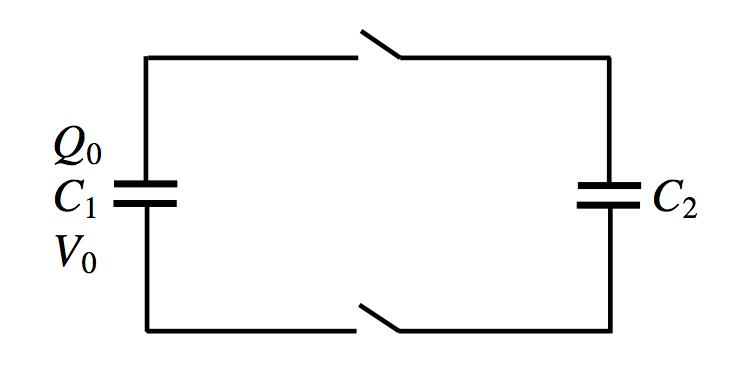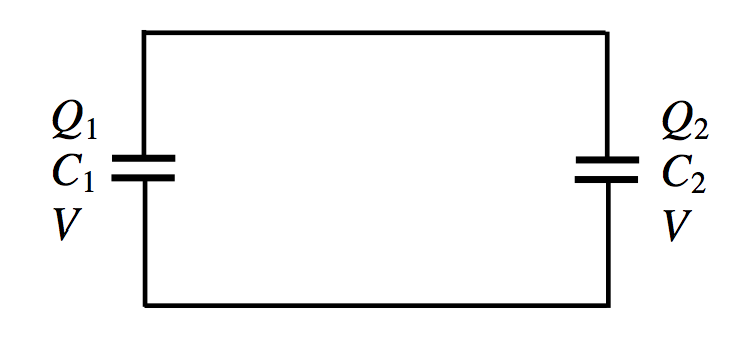$$\require{cancel}$$

# 5.13: Sharing a Charge Between Two Capacitors

$$\newcommand{\vecs}{\overset { \rightharpoonup} {\mathbf{#1}} }$$ $$\newcommand{\vecd}{\overset{-\!-\!\rightharpoonup}{\vphantom{a}\smash {#1}}}$$$$\newcommand{\id}{\mathrm{id}}$$ $$\newcommand{\Span}{\mathrm{span}}$$ $$\newcommand{\kernel}{\mathrm{null}\,}$$ $$\newcommand{\range}{\mathrm{range}\,}$$ $$\newcommand{\RealPart}{\mathrm{Re}}$$ $$\newcommand{\ImaginaryPart}{\mathrm{Im}}$$ $$\newcommand{\Argument}{\mathrm{Arg}}$$ $$\newcommand{\norm}{\| #1 \|}$$ $$\newcommand{\inner}{\langle #1, #2 \rangle}$$ $$\newcommand{\Span}{\mathrm{span}}$$ $$\newcommand{\id}{\mathrm{id}}$$ $$\newcommand{\Span}{\mathrm{span}}$$ $$\newcommand{\kernel}{\mathrm{null}\,}$$ $$\newcommand{\range}{\mathrm{range}\,}$$ $$\newcommand{\RealPart}{\mathrm{Re}}$$ $$\newcommand{\ImaginaryPart}{\mathrm{Im}}$$ $$\newcommand{\Argument}{\mathrm{Arg}}$$ $$\newcommand{\norm}{\| #1 \|}$$ $$\newcommand{\inner}{\langle #1, #2 \rangle}$$ $$\newcommand{\Span}{\mathrm{span}}$$$$\text{FIGURE V.14}$$

We have two capacitors. $$\text{C}_2$$ is initially uncharged. Initially, $$\text{C}_1$$ bears a charge $$Q_0$$ and the potential difference across its plates is $$V_0$$, such that

$Q_0=C_1V_0,$

and the energy of the system is

$U_0=\frac{1}{2}C_1V_0^2.$

We now close the switches, so that the charge is shared between the two capacitors:$$\text{FIGURE V.15}$$

The capacitors $$\text{C}_1$$ and $$\text{C}_2$$ now bear charges $$Q_1$$ and $$Q_2$$ such that $$Q_0 = Q_1 + Q_2$$ and

$Q_1=\frac{C_1}{C_1+C_2}Q_0\quad \text{ and }\quad Q_2=\frac{C_2}{C_1+C_2}Q_0.$

The potential difference across the plates of either capacitor is, of course, the same, so we can call it $$V$$ without a subscript, and it is easily seen, by applying $$Q = CV$$ to either capacitor, that

$V=\frac{C_1}{C_1+C_2}V_0.$

We can now apply $$U=\frac{1}{2}CV^2$$ to each capacitor in turn to find the energy stored in each. We find for the energies stored in the two capacitors:

$U_1=\frac{C_1^3V_0^2}{2(C_1+C_2)^2}\quad \text{ and }\quad U_2=\frac{C_2C_1^2V_0^2}{2(C_1+C_2)^2}.$

The total energy stored in the two capacitors is the sum of these, which is

$U=\frac{C_1^2V_0^2}{2(C_1+C_2)},$

which can also be written

$U=\frac{C_1}{C_1+C_2}U_0.$

Surprise, surprise! The energy stored in the two capacitors is less than the energy that was originally stored in $$\text{C}_1$$. What has happened to the lost energy?

A perfectly reasonable and not incorrect answer is that it has been dissipated as heat in the connecting wires as current flowed from one capacitor to the other. However, it has been found in low temperature physics that if you immerse certain metals in liquid helium they lose all electrical resistance and they become superconductive. So, let us connect the capacitors with superconducting wires. Then there is no dissipation of energy as heat in the wires – so the question remains: where has the missing energy gone?

Well, perhaps the dielectric medium in the capacitors is heated? Again this seems like a perfectly reasonable and probably not entirely incorrect answer. However, my capacitors have a vacuum between the plates, and are connected by superconducting wires, so that no heat is generated either in the dielectric or in the wires. Where has that energy gone?

This will have to remain a mystery for the time being, and a topic for lunchtime conversation. In a later chapter I shall suggest another explanation.

This page titled 5.13: Sharing a Charge Between Two Capacitors is shared under a CC BY-NC 4.0 license and was authored, remixed, and/or curated by Jeremy Tatum via source content that was edited to the style and standards of the LibreTexts platform; a detailed edit history is available upon request.